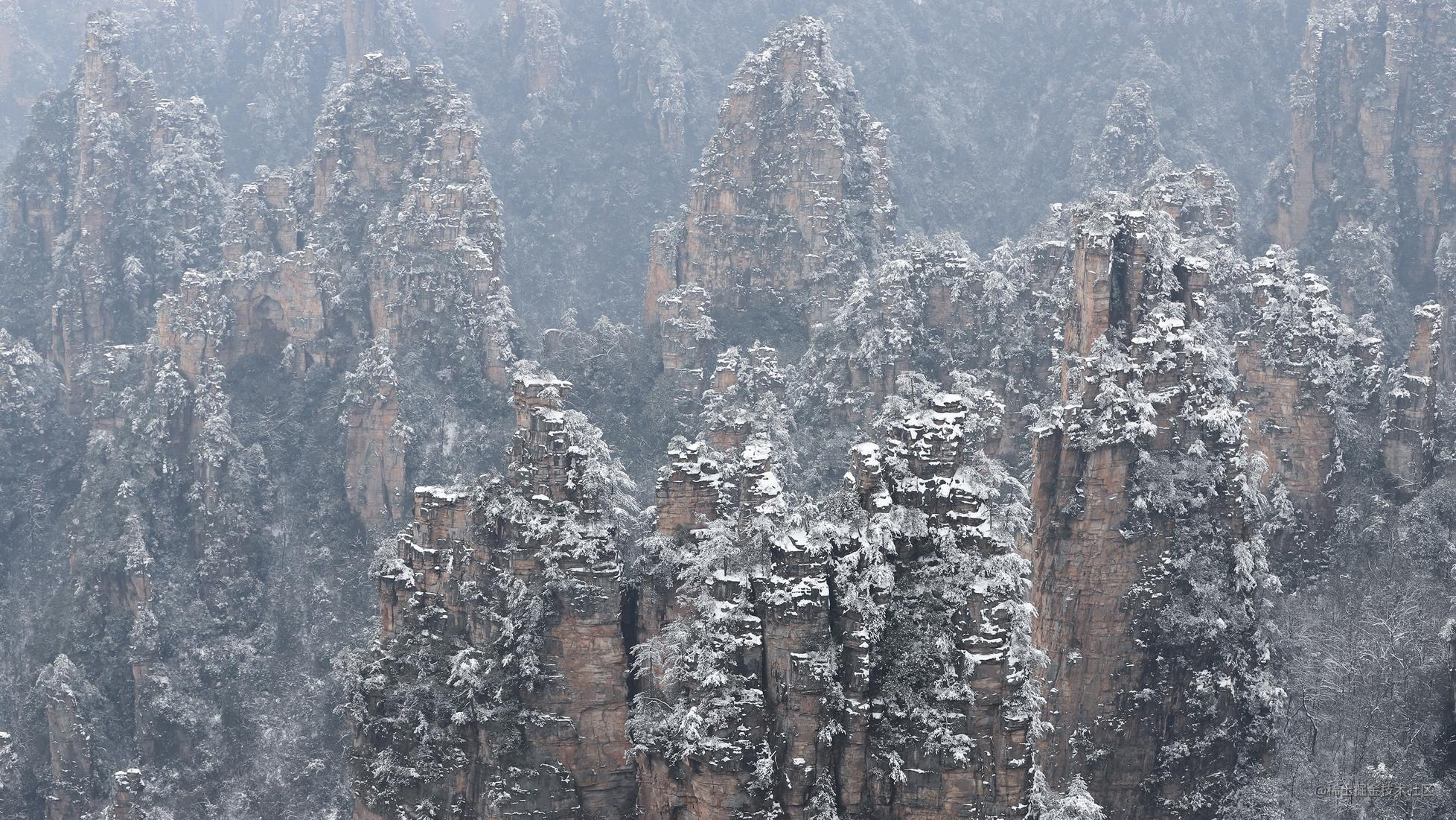# 自带美感的贝塞尔曲线原理与实战——Android高级UI

## 二、贝塞尔曲线的绘制规则

### 1、一阶贝塞尔曲线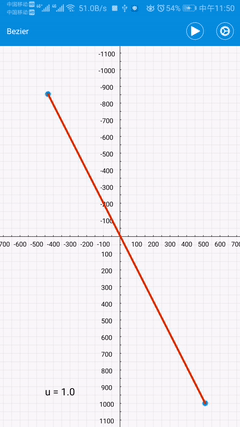### 2、二阶贝塞尔曲线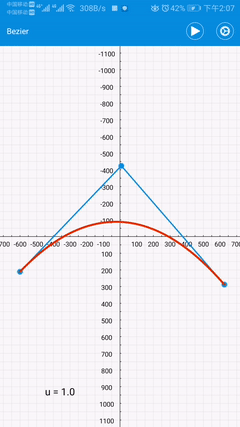AD/AB = BE/BC = u(比例值)

DF/DE = u(比例值)

#### 从上面的三步中，我们可以得出如下三条结论：

• 一阶的基线从二阶得来，二阶的基线从三阶得来（待会会继续讲三阶，稍安勿躁），推而广之，n阶的基线便从（n+1）阶得来
• 除了最高阶的控制点是固定的，降阶过程中的控制点全都是按 比例值u 进行取点。所以在二阶的例子中，我们可以得出以下这样一个等式
AD/AB = BE/BC = DF/DE = u(比例值)

• 贝塞尔曲线最终的路径是由 一阶基线 上的游走的红色小点形成的；

### 3、三阶贝塞尔曲线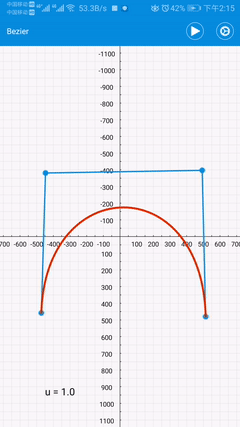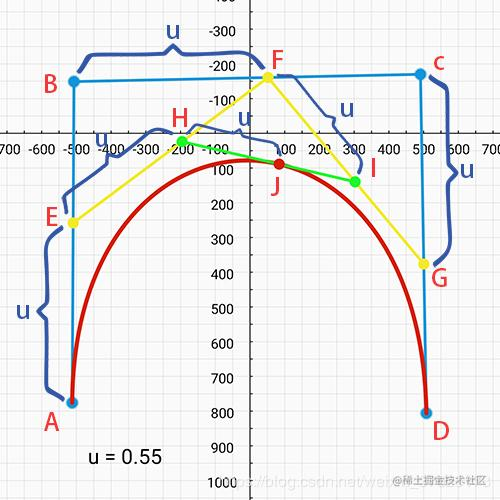#### 值得一提

AE/AB = BF/BC = CG/CD = EH/EF = FI/FG = HJ/HI = 比例值u

### 4、七阶贝塞尔曲线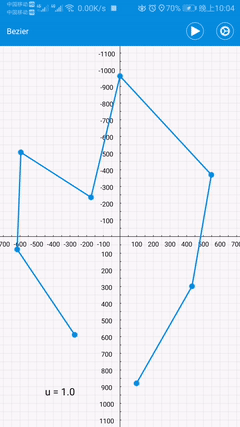## 三、在canvas中如何绘制贝塞尔曲线

### 1、二阶贝塞尔曲线

public void quadTo(float x1, float y1, float x2, float y2)

#### 如何使用？

// 初始化 路径对象
Path path = new Path();
// 移动至第一个控制点 A(ax,ay)
path.moveTo(ax, ay);
// 填充二阶贝塞尔曲线的另外两个控制点 B(bx,by) 和 C(cx,cy)，切记顺序不能变
// 将 贝塞尔曲线 绘制至画布
canvas.drawPath(path, paint);

### 2、三阶贝塞尔曲线

public void cubicTo(float x1, float y1, float x2, float y2, float x3, float y3)

// 初始化 路径对象
Path path = new Path();
// 移动至第一个控制点 A(ax,ay)
path.moveTo(ax, ay);
// 填充三阶贝塞尔曲线的另外三个控制点：
// B(bx,by) C(cx,cy) D(dx,dy) 切记顺序不能变
path.cubicTo(bx, by, cx, cy, dx, dy);
// 将 贝塞尔曲线 绘制至画布
canvas.drawPath(path, paint);

### 3、多阶贝塞尔曲线

D点的x轴坐标：dx = ax + (bx-ax) * u  = (1-u) * ax + u * bx   (u ∈ [0,1])
D点的y轴坐标：dy = ay + (by-ay) * u  = (1-u) * ay + u * by   (u ∈ [0,1])

E点的x轴坐标：ex = bx + (cx-bx) * u  = (1-u) * bx + u * cx   (u ∈ [0,1])
E点的y轴坐标：ey = by + (cy-by) * u  = (1-u) * by + u * cy   (u ∈ [0,1])

F点的x轴坐标：fx = dx + (ex-dx) * u  = (1-u) * dx + u * ex   (u ∈ [0,1])
F点的y轴坐标：fy = dy + (ey-dy) * u  = (1-u) * dy + u * ey   (u ∈ [0,1])

#### 推导公式

P0k = (1-u) * P0k-1 + u * P1k-1

Tips: x轴 和 y轴 的坐标计算公式是一样，所以我们这里就使用 x轴 作为代表，方便讲解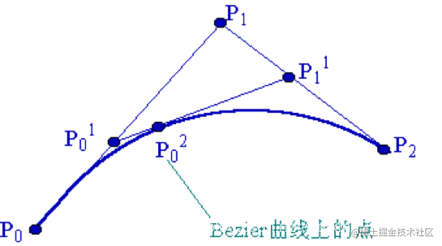1、k 表示阶数，当 k=n 时，即相当于前面demo所讲的一阶控制点；当 k=0 时，表示最高阶的控制点，即我们程序猿最初给定的那几个控制点；

2、 i 表示点的下标，这个只是为了便于区分，可参照上面的图进行带入理解；

3、u 表示比例值

/**
* 构建贝塞尔曲线，具体点数由 参数frame 决定
*
* @param controlPointList 控制点的坐标
* @param frame            帧数
* @return
*/
public static List<PointF> buildBezierPoint(List<PointF> controlPointList,
int frame) {
List<PointF> pointList = new ArrayList<>();

// 此处注意，要用1f，否则得出结果为0
float delta = 1f / frame;

// 阶数，阶数=绘制点数-1
int order = controlPointList.size() - 1;

// 循环递增
for (float u = 0; u <= 1; u += delta) {
pointList.add(new PointF(BezierUtils.calculatePointCoordinate(BezierUtils.X_TYPE, u, order, 0, controlPointList),
BezierUtils.calculatePointCoordinate(BezierUtils.Y_TYPE, u, order, 0, controlPointList)));
}

return pointList;

}

/**
* 计算坐标 [贝塞尔曲线的核心关键]
*
* @param u                当前的比例
* @param k                阶数
* @param p                当前坐标（具体为 x轴 或 y轴）
* @param controlPointList 控制点的坐标
* @return
*/
public static float calculatePointCoordinate(@IntRange(from = X_TYPE, to = Y_TYPE) int type,
float u,
int k,
int p,
List<PointF> controlPointList) {

/**
* 公式解说：（p表示坐标点，后面的数字只是区分）
* 场景：有一条线p1到p2，p0在中间，求p0的坐标
*      p1◉--------○----------------◉p2
*            u    p0
*
* 公式：p0 = p1+u*(p2-p1) 整理得出 p0 = (1-u)*p1+u*p2
*/

// 一阶贝塞尔，直接返回
if (k == 1) {

float p1;
float p2;

// 根据是 x轴 还是 y轴 进行赋值
if (type == X_TYPE) {
p1 = controlPointList.get(p).x;
p2 = controlPointList.get(p + 1).x;
} else {
p1 = controlPointList.get(p).y;
p2 = controlPointList.get(p + 1).y;
}

return (1 - u) * p1 + u * p2;

} else {

/**
* 这里应用了递归的思想：
* 1阶贝塞尔曲线的端点 依赖于 2阶贝塞尔曲线
* 2阶贝塞尔曲线的端点 依赖于 3阶贝塞尔曲线
* ....
* n-1阶贝塞尔曲线的端点 依赖于 n阶贝塞尔曲线
*
* 1阶贝塞尔曲线 则为 真正的贝塞尔曲线存在的点
*/
return (1 - u) * calculatePointCoordinate(type, u, k - 1, p, controlPointList)
+ u * calculatePointCoordinate(type, u, k - 1, p + 1, controlPointList);

}

}

## 四、实战

### 1、圆变心

#### 效果图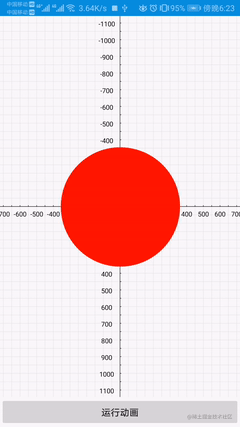#### 准备零件

（1）圆 此圆非彼圆，我们不能借助canvas直接使用drawCircle进行绘制，因为这样的圆我们无法控制。那要如何处理呢？当然是用贝塞尔曲线画圆，因为这样一来这个 “圆”的控制点 就全都在我们的可控范围内，因为我持有了这些控制点就能进行坐标的变动，进而改变曲线的形状。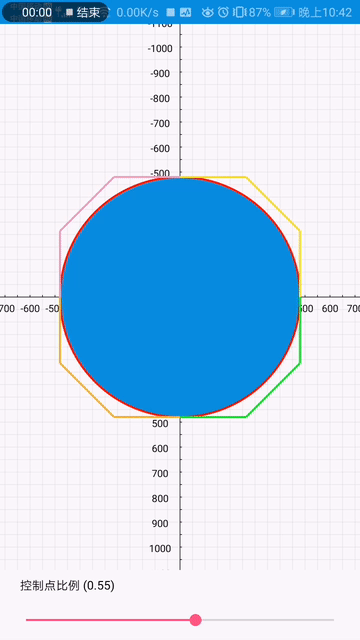$控制点比例 =\frac{AB}{ED} = \frac{CD}{AE}$

E为圆心，AE和ED为半径，即AE=ED；所以AB=CD;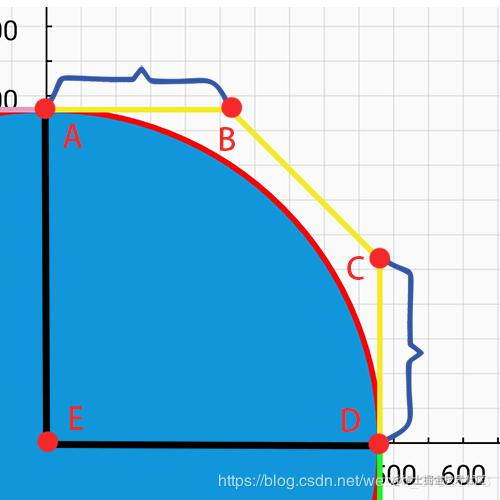（2）心 心要如何绘制呢？小盆友这里给出一个小工具，我们可以通过自行拽动来获取需要的图形，然后打印坐标（单位dp），拿到坐标了就可以为所欲为了。工具效果图如下，我们以拽动一个圆为例：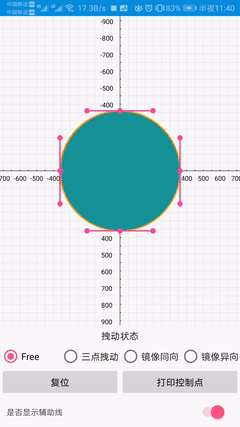（3）弹性效果公式 终于到最后一个零件啦，弹性效果公式可以从一个网站尝试得出

inloop.github.io/interpolato…

float x = (float) animation.getAnimatedValue();
float factor = 0.15f;
double value = Math.pow(2, -10 * x) * Math.sin((x - factor / 4) * (2 * Math.PI) / factor) + 1;

### 2、乘风破浪的小船

#### 效果图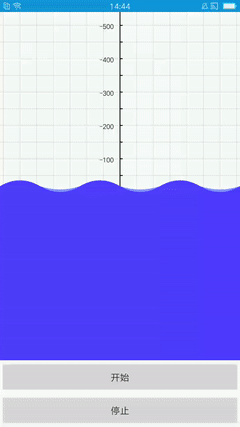#### 大致思路

（1）绘制蓝色的波浪、浅蓝色的波浪和小船的轨迹，这里使用的是二阶贝塞尔曲线 （2）将蓝色和浅蓝色的波浪的波浪绘制至canvas，但偏移量不同。 （3）使用 PathMeasure 测量小船轨迹，同时改变的坐标和方向。

### 3、粘性小红点

#### 效果图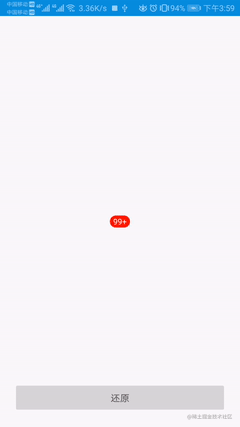## 五、写在最后